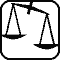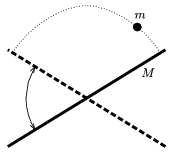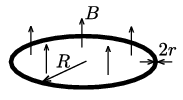Mathematical and Physical Journal
for High Schools
Issued by the MATFUND Foundation
 Already signed up? New to KöMaL?

# Exercises and problems in PhysicsOctober 2003## Experimental problem

M. 245. Prepare sugar solutions of the same mass but of different concentrations. Measure how much time it takes to heat the solutions with an immersion heater to a certain, not too high final temperature from a given initial temperature. Determine how the specific heat of a solution depends on its concentration. (6 points)## Theoretical problems

It is allowed to send solutions for any number of problems, but final scores of students of grades 9-12 are computed from the 5 best score in each month. Final scores of students of grades 1-8 are computed from the 3 best scores in each month.

P. 3642. A passenger, being somewhat late, runs after the bus with a velocity of 5 m/s. He is at an 11 m distance from the bus when it starts off with an acceleration of 1 m/s2. Where and when does he catch the bus up? (3 points)

P. 3643. Two resistances, a 60 ohm and an unknown one are connected to a power source in a series arrangement. This way the power of the unknown resistance is 60 W. What is the voltage of the power source at the least? (4 points)

P. 3644. In three different tanks, there is air of temperatures 300 K, 500 K and 720 K, respectively. Allowing heat exchange between the gases of 300 K and 500 K the common temperature is 420 K. Allowing heat exchange between the gases of 300 K and 720 K the common temperature is 500 K. To what common temperature the 500 K and 720 K gases would settle together? What is the ratio of the internal energies of the gases in the beginning? (4 points)

P. 3645. Inert gas of a certain mass expands under constant pressure from a volume of V1 to V2. In the meantime it does work of 13 kJ in the expansion. How much heat would it absorb if the pressure were 35% higher? (4 points)

P. 3646. A homogeneous bar of mass M waggles (rotates uniformly in alternating directions) to and from around the axis going through its center, while a ball of mass m bounces between the ends of the bar as shown in the figure. The angular velocity of the bar is $\displaystyle \pm$2$\displaystyle \pi$/3 s-1 and the period of the movement of the ball is 1 second. (The mechanical loss and the duration of the collisions are negligible.) a) What is the velocity of the ball at the highest point of its orbit? b) How long is the bar? c) What is the m/M ratio? (5 points)P. 3647. In country NOSI'' the system of units of measure deviates in a few points from the SI system. The time unit is one hundred-thousandth of the day, and the unit of the electric charge is the hundred-thousandth part of the charge of as many electrons as Avogadro's number. What is the value of the gravitational acceleration and the constant k in Coulomb's law in this country? (4 points)

P. 3648. We make a loop of radius R by bending a cylindrical shape conductor cable of radius r$\displaystyle \ll$R and put it into a magnetic field of induction B perpendicular to its plane. Then we uniformly decrease B to zero in $\displaystyle \tau$ time. How much does this circular conductor heat up if its conductivity is $\displaystyle \sigma$, its density is $\displaystyle \varrho$, and its coefficient of specific heat is c? (4 points)P. 3649. The intended period of revolution of a space probe around comet Virtanen is 6 days. Let us consider the comet as a loose snowball of a diameter of 800 m with a density of 10 kg/m3. a) At what distance should the probe revolve? b) What is the revolution time of the comet around the Sun if it orbits between the orbits of the Earth and Jupiter? (5 points)

P. 3650. How thick an iron plate should be to ensure that any line perpendicular to its surface would go through an atomic nucleus? (4 points)

P. 3651. In 1851 in the Paris Pantheon Foucault (1819-1868) the French physicist proved that the Earth rotates with a 67 m long pendulum of a mass of 28 kg. Even if he hadn't simply let the pendulum loose, but had rather pushed it perpendicularly to its original displacement with the proper velocity to ensure a uniform circular motion (conical pendulum), he could have proven Earth's rotation. How? (5 points)